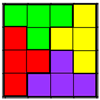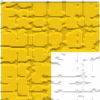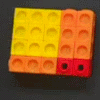Seeing Is Believing

Some problems become much clearer when you find a good image to represent them, and some mathematical results can be proved beautifully with just a simple diagram.

The Seeing is Believing pathway on wild.maths.org offers students situations where they can draw their own diagrams as well as using our images to discover relationships and make connections.

The collection of related NRICH tasks below are ideal for teachers who want to promote creativity in the classroom. They are designed for classroom use, with accompanying Teachers' Notes and Resources.Tetrafit

Age 7 to 11 Challenge Level:

A tetromino is made up of four squares joined edge to edge. Can this tetromino, together with 15 copies of itself, be used to cover an eight by eight chessboard?The Remainders Game

Age 7 to 14 Challenge Level:

Play this game and see if you can figure out the computer's chosen number.Remainders

Age 7 to 14 Challenge Level:

I'm thinking of a number. My number is both a multiple of 5 and a multiple of 6. What could my number be?Summing Consecutive Numbers

Age 11 to 14 Challenge Level:

15 = 7 + 8 and 10 = 1 + 2 + 3 + 4. Can you say which numbers can be expressed as the sum of two or more consecutive integers?What Numbers Can We Make?

Age 11 to 14 Challenge Level:

Imagine we have four bags containing a large number of 1s, 4s, 7s and 10s. What numbers can we make?Reflecting Squarely

Age 11 to 14 Challenge Level:

In how many ways can you fit all three pieces together to make shapes with line symmetry?Diminishing Returns

Age 11 to 14 Challenge Level:

How much of the square is coloured blue? How will the pattern continue?Picturing Triangular Numbers

Age 11 to 14 Challenge Level:

Triangular numbers can be represented by a triangular array of squares. What do you notice about the sum of identical triangle numbers?Sieve of Eratosthenes

Age 11 to 14 Challenge Level:

Follow this recipe for sieving numbers and see what interesting patterns emerge.Picturing Square Numbers

Age 11 to 14 Challenge Level:

Square numbers can be represented as the sum of consecutive odd numbers. What is the sum of 1 + 3 + ..... + 149 + 151 + 153?Seven Squares

Age 11 to 14 Challenge Level:

Watch these videos to see how Phoebe, Alice and Luke chose to draw 7 squares. How would they draw 100?Always a Multiple?

Age 11 to 14 Challenge Level:

Think of a two digit number, reverse the digits, and add the numbers together. Something special happens...Squares in Rectangles

Age 11 to 14 Challenge Level:

A 2 by 3 rectangle contains 8 squares and a 3 by 4 rectangle contains 20 squares. What size rectangle(s) contain(s) exactly 100 squares? Can you find them all?Cubes Within Cubes Revisited

Age 11 to 14 Challenge Level:

Imagine starting with one yellow cube and covering it all over with a single layer of red cubes, and then covering that cube with a layer of blue cubes. How many red and blue cubes would you need?Triominoes

Age 11 to 14 Challenge Level:

A triomino is a flat L shape made from 3 square tiles. A chess board is marked into squares the same size as the tiles and just one square, anywhere on the board, is coloured red. Can you cover the board with trionimoes so that only the square is exposed?Marbles in a Box

Age 11 to 16 Challenge Level:

How many winning lines can you make in a three-dimensional version of noughts and crosses?Steel Cables

Age 14 to 16 Challenge Level:

Some students have been working out the number of strands needed for different sizes of cable. Can you make sense of their solutions?Double Trouble

Age 14 to 16 Challenge Level:

Simple additions can lead to intriguing results...Age 14 to 16 Challenge Level:

Can you find out what is special about the dimensions of rectangles you can make with squares, sticks and units?Partly Painted Cube

Age 14 to 16 Challenge Level:

Jo made a cube from some smaller cubes, painted some of the faces of the large cube, and then took it apart again. 45 small cubes had no paint on them at all. How many small cubes did Jo use?Painted Cube

Age 14 to 16 Challenge Level:

Imagine a large cube made from small red cubes being dropped into a pot of yellow paint. How many of the small cubes will have yellow paint on their faces?L-triominoes

Age 14 to 16 Challenge Level:

L triominoes can fit together to make larger versions of themselves. Is every size possible to make in this way?Picture Story

Age 14 to 16 Challenge Level:

Can you see how this picture illustrates the formula for the sum of the first six cube numbers?Attractive Tablecloths

Age 14 to 16 Challenge Level:

Charlie likes tablecloths that use as many colours as possible, but insists that his tablecloths have some symmetry. Can you work out how many colours he needs for different tablecloth designs?Plus Minus

Age 14 to 16 Challenge Level:

Can you explain the surprising results Jo found when she calculated the difference between square numbers?Mystic Rose

Age 14 to 16 Challenge Level:

Use the animation to help you work out how many lines are needed to draw mystic roses of different sizes.AMGM

Age 14 to 16 Challenge Level:

Can you use the diagram to prove the AM-GM inequality?